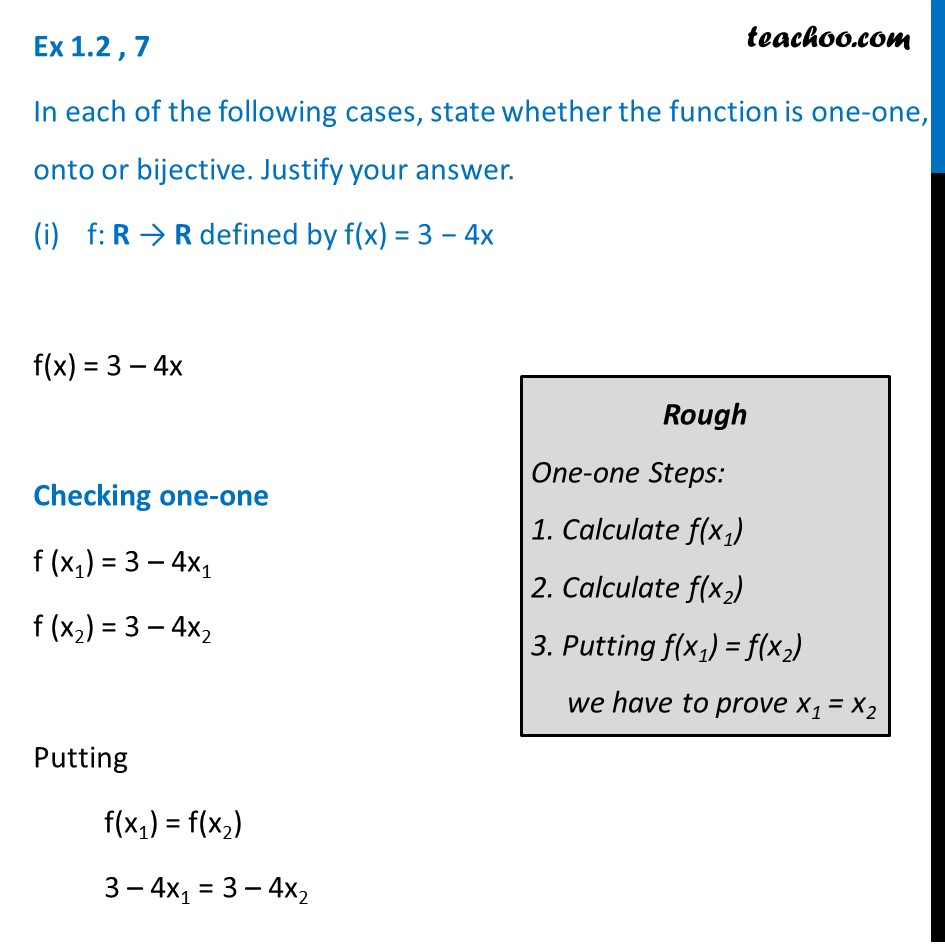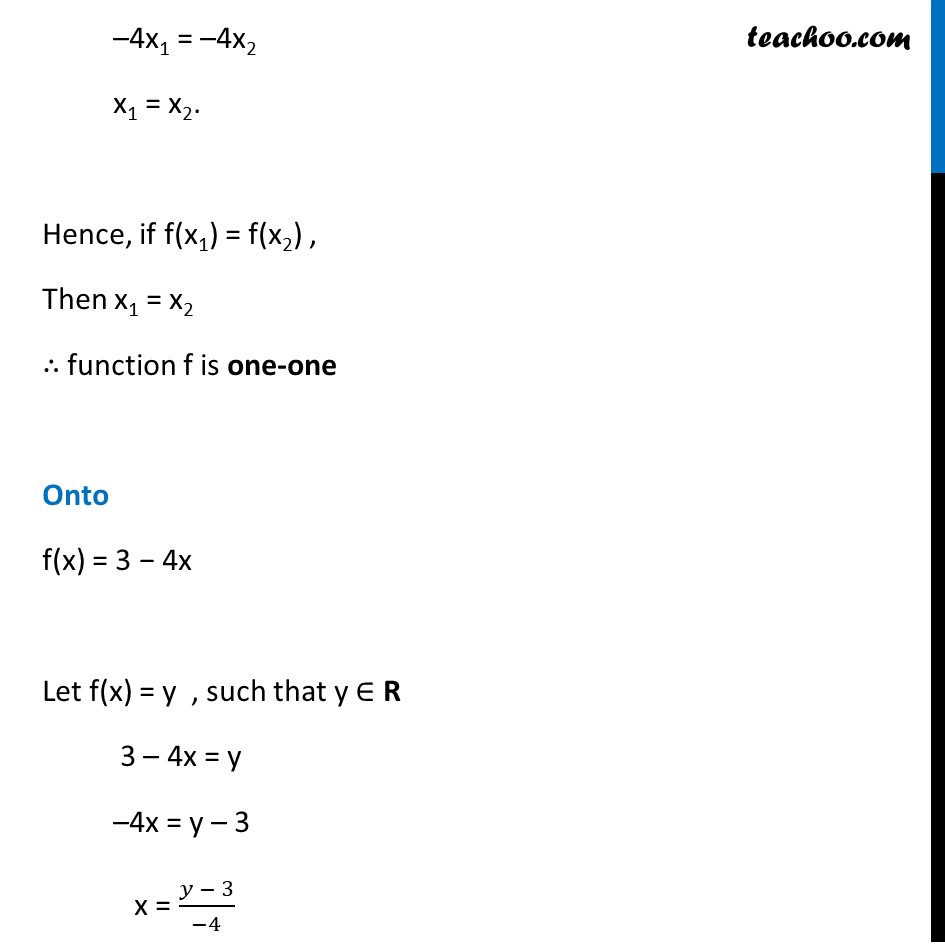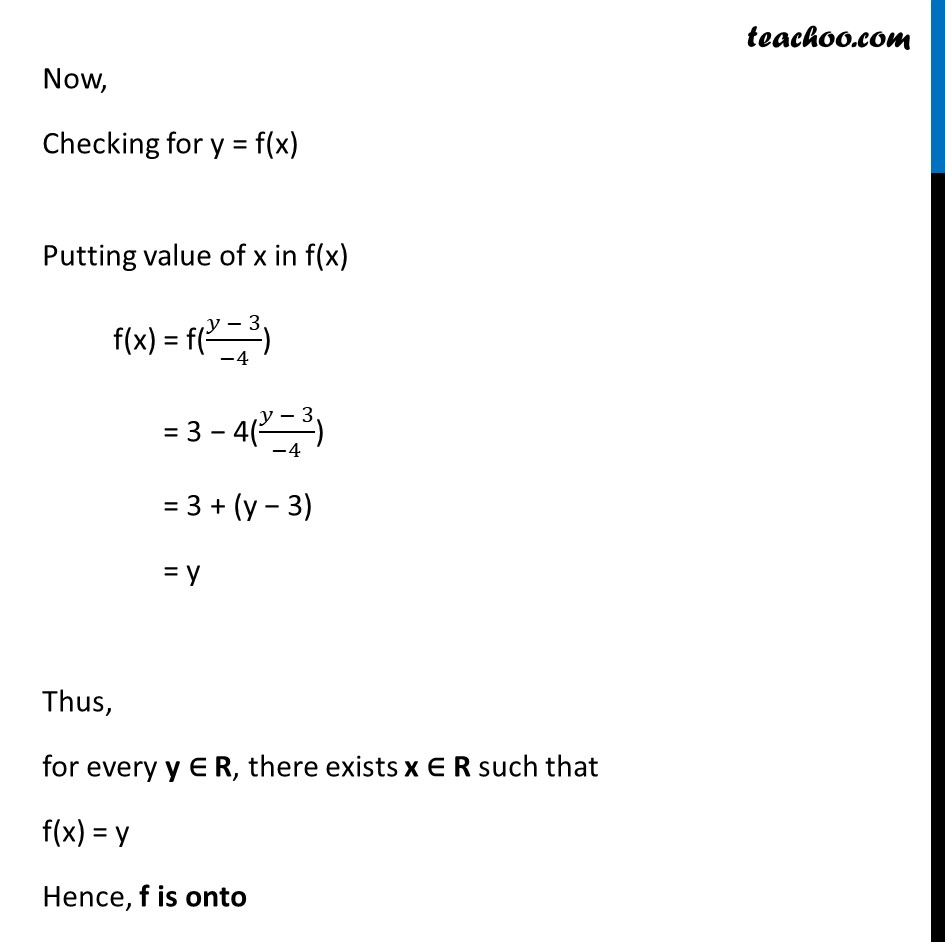To prove one-one & onto (injective, surjective, bijective)

Chapter 1 Class 12 Relation and Functions
Concept wiseLearn in your speed, with individual attention - Teachoo Maths 1-on-1 Class

### Transcript

Ex 1.2 , 7 In each of the following cases, state whether the function is one-one, onto or bijective. Justify your answer. f: R → R defined by f(x) = 3 − 4x f(x) = 3 – 4x Checking one-one f (x1) = 3 – 4x1 f (x2) = 3 – 4x2 Putting f(x1) = f(x2) 3 – 4x1 = 3 – 4x2 Rough One-one Steps: 1. Calculate f(x1) 2. Calculate f(x2) 3. Putting f(x1) = f(x2) we have to prove x1 = x2 –4x1 = –4x2 x1 = x2. Hence, if f(x1) = f(x2) , Then x1 = x2 ∴ function f is one-one Onto f(x) = 3 − 4x Let f(x) = y , such that y ∈ R 3 – 4x = y –4x = y – 3 x = (𝑦 − 3)/(−4) Now, Checking for y = f(x) Putting value of x in f(x) f(x) = f((𝑦 − 3)/(−4)) = 3 − 4((𝑦 − 3)/(−4)) = 3 + (y − 3) = y Thus, for every y ∈ R, there exists x ∈ R such that f(x) = y Hence, f is onto